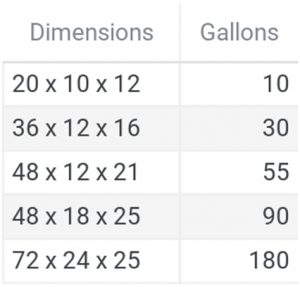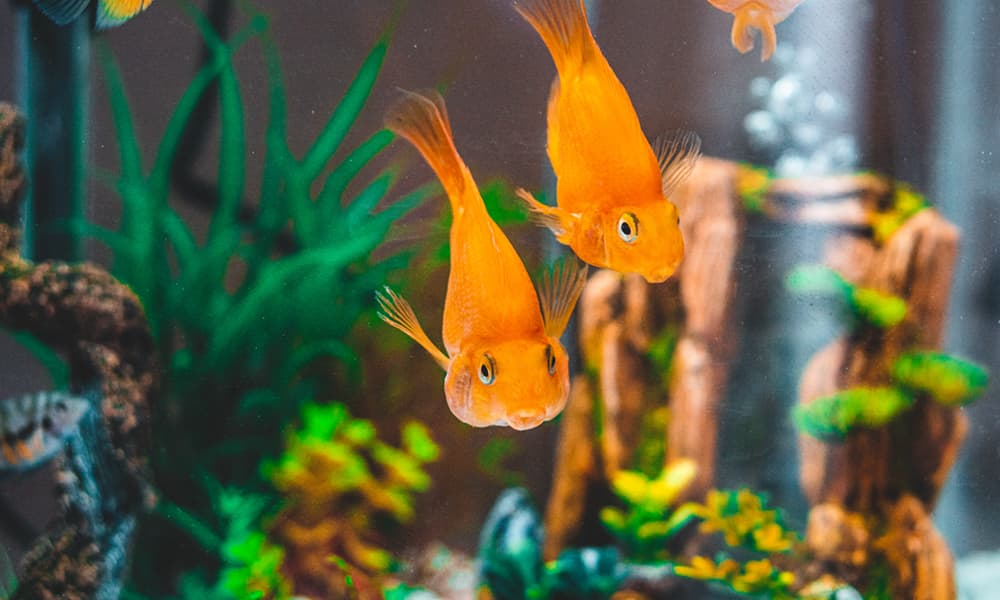# Aquarium Size Chart – How Can I Figure Out How Much Water My Fish Tank Holds?

If you buy your aquarium in a pet store or order one online, it’s easy to figure out how much water it holds. This information, like aquarium dimensions and water capacity, is usually found right on the packaging or in the manual that comes with the fish tank.

But what do you do if you buy a fish tank second hand or if someone gives you a large tank and you’re not entirely sure how much water it holds? Knowing the total water volume of your tank is essential for choosing the right canister filter, lighting, and heater, as well as making sure you’re not overstocking it with fish.

When you don’t have the original packaging, there are a few ways to figure out your aquarium dimensions and its gallon capacity. Let’s take a look.

## Standard Aquarium Size Chart

The first thing you have to do is to measure the dimensions of your new aquarium. Fish tanks come in a range of shapes and sizes, but there are standard large and small tank sizes. This aquarium size chart tells you the gallon capacity these common sizes can hold:## What If My Aquarium Has Different Dimensions?

If you measured the dimensions of your aquarium and they don’t fit one of the categories above, you’ll have to do some math.

### Rectangular Tanks

If you have a rectangular tank, use a measuring tape to get the length, width, and height. For accuracy, measure from the outside of the tank and then subtract the width of the tank walls. So, if your tank is 13 inches long but the glass is ½ inch thick, you will subtract one inch (½ inch for each side) for a length of 12 inches.

Multiply the final length, width, and height. This gives you the total volume of your tank in cubic inches. Using the dimension for a 30-gallon tank above as an example, we would multiply 36 x 12 x 16 inches, which is 6,912 cubic inches.

To convert cubic inches to gallons, divide by 231; so, 6,912 divided by 231 is 29.9 or 30 gallons.

### Cylindrical TanksTo find the volume of a cylinder, you need to measure the height and radius. If you don’t remember from high school geometry, the radius is the distance from the center of the circle to the edge. The easiest way to do this is to measure across the width of the tank (again, accounting for the thickness of the walls) to get the diameter and then dividing by two.

Then, square the radius and multiply it by pi, or 3.14. Finally, multiply the result by the height of the aquarium to get the volume in cubic inches.

So, let’s say you have a cylindrical tank that’s 12 inches in diameter and 24 inches tall, after accounting for the wall thickness.

Divide the diameter in half to get the radius, which is 6 inches. Next, square that number by multiplying it by itself to get 36, then multiply that by pi. So, 36 times 3.14 is about 77.

To get the volume in cubic inches, multiply the result by the height, so 77 times 24 or 1,848. To convert to gallons, divide by 231 for a tank volume of 8 gallons.

## How Much Water Your Tank Holds

Now that you know exactly how much water your tank can hold, it’s worth mentioning that your aquarium is not going to hold this much water. Why? Because anything you put in your tank will take up some of the volume, including substrate, plants, and decorations. Generally, about 10 percent of the volume of your tank will be taken up by something other than water.

## Why It’s Important to Know Aquarium Size

It’s impossible to properly set up an aquarium without knowing how much water it holds. You need this information to make sure your filter is powerful enough to cycle the tank and that the lights and heater are the right sizes for the tank.

You also have to know the types of fish that are appropriate for the size of your aquarium. Putting too many fish into a tank will likely end in disaster, so be sure you know what you’re working with before you add any fish friends.